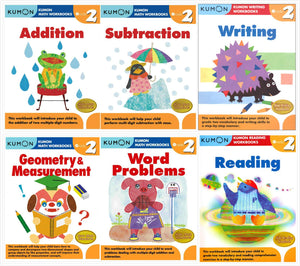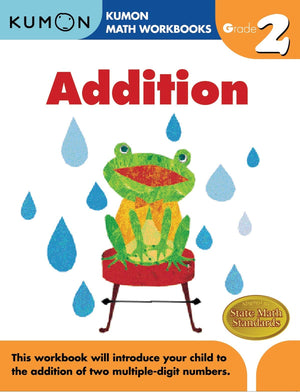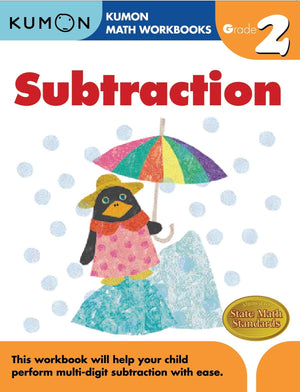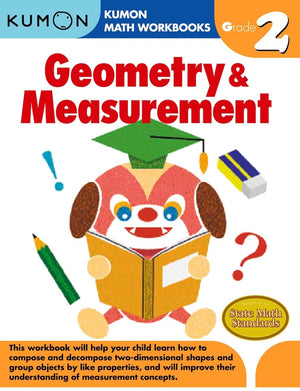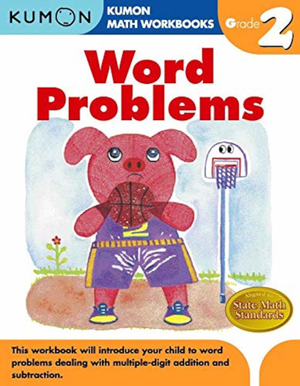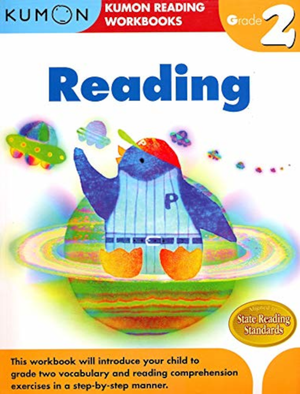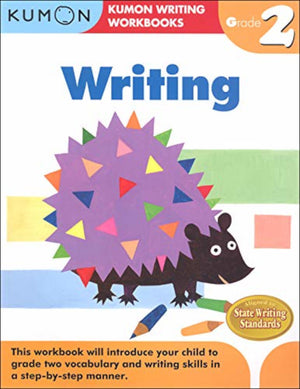# Kumon Math and More Workbooks Grade 2 (Set of 6)

Regular price \$48.95
Unit price  per

Kumon Math and More Workbooks Grade 2 (Set of 6): Addition, Subtraction, Writing, Geometry & Measurement, Word Problems, and Reading

This Kumon set includes the following 6 workbooks for second grade: Addition, Subtraction, Writing, Geometry & Measurement, Word Problems, and Reading. Challenging curriculum that makes problem solving, mathematics skills, and homeschool easy! Addition: Children progress to two-digit addition while learning the commutative property of addition- an important foundation for future math success. Problems incorporate both horizontal and vertical form addition, which prepares children for practice with three and four-digit addition. Subtraction: helps your child subtract three-digit numbers with confidence. Children will learn to regroup (borrow) when subtracting, which equips them with the tools to subtract three-digit numbers. Vertical subtraction is included along with patterning and sequencing activities. Writing: Topics Covered in this Book: Vocabulary, Nouns, pronouns, verbs, adverbs, and adjectives, Irregular verbs, Contractions, Subjects and predicates, Punctuation, Writing a story, Aligned to State Standards. Geometry & Measurement: activities progress gradually, so your child can confidently work with triangles and quadrilaterals, one, five, ten, and twenty-dollar bills, and time to the nearest five minutes. Compare and order numbers -Identify, recognize, name, sort and compare basic two- and three-dimensional shapes. Use non-standard units to measure and compare concrete shapes. Coins (penny, nickel, dime, quarter). Telling time (to the hour and a half hour). Word problems: the basics of answering word problems with large numbers. Length and weight word problems give children real world addition and subtraction applications. Through our gradual progression of activities, your child will advance to tables and graphs with ease.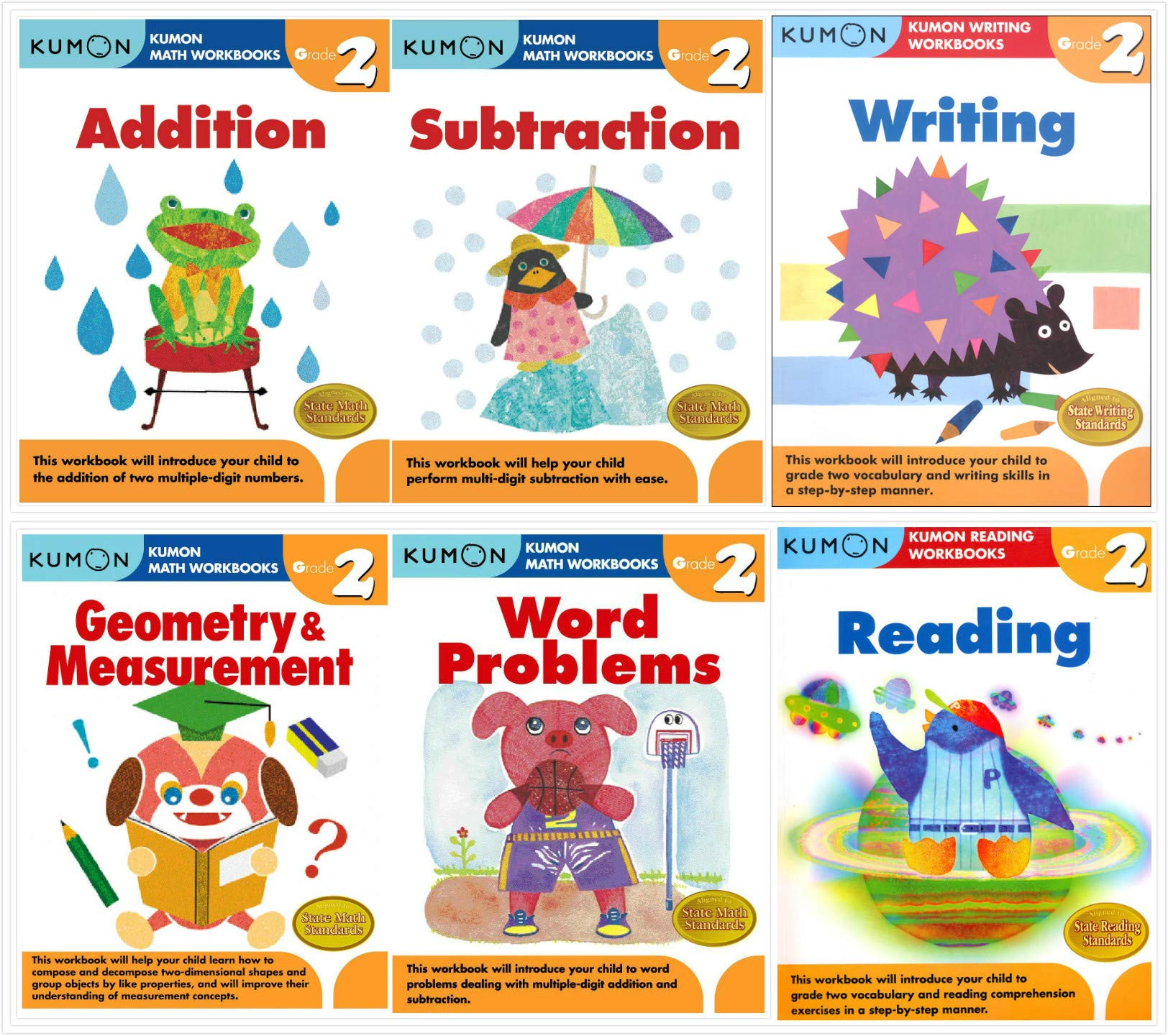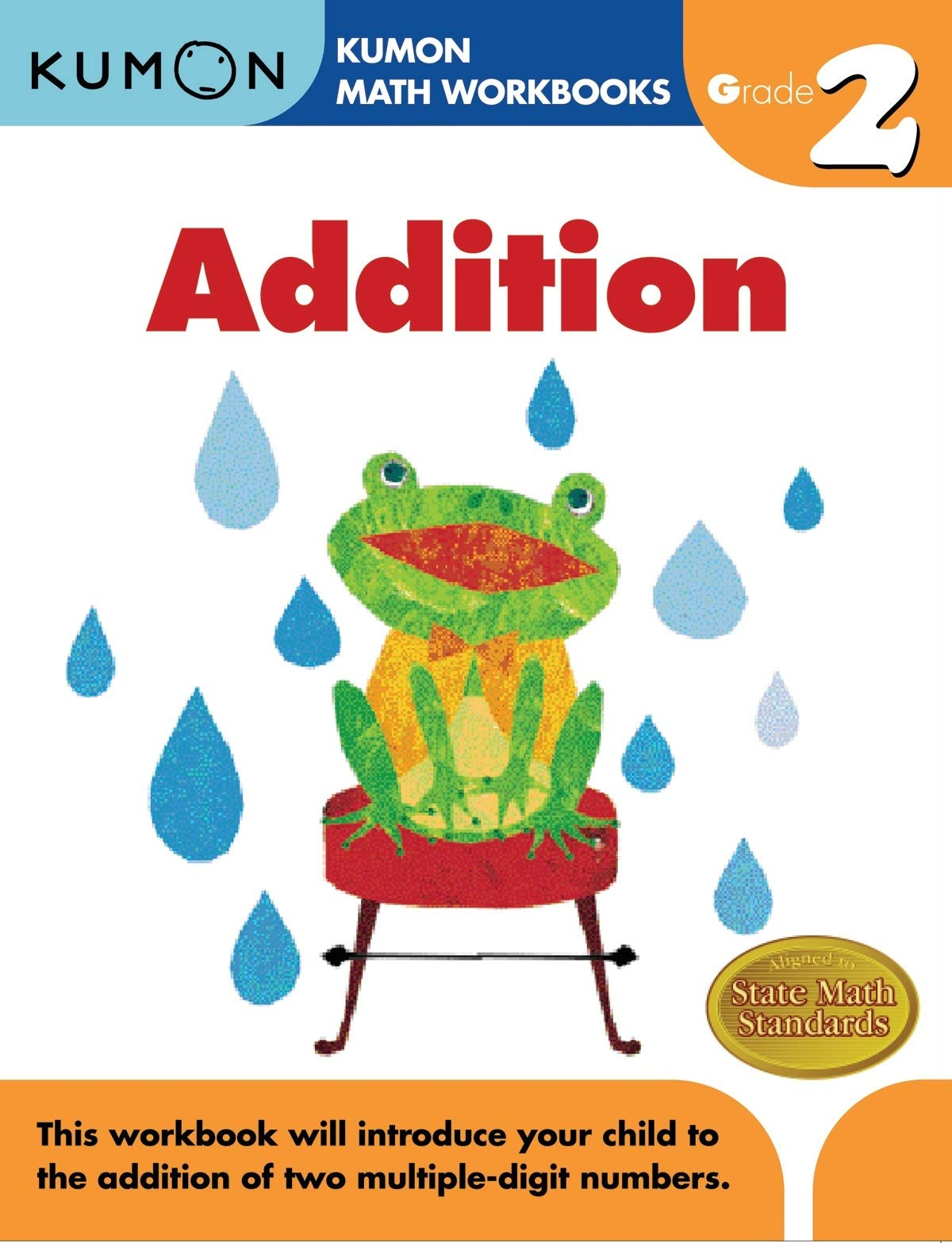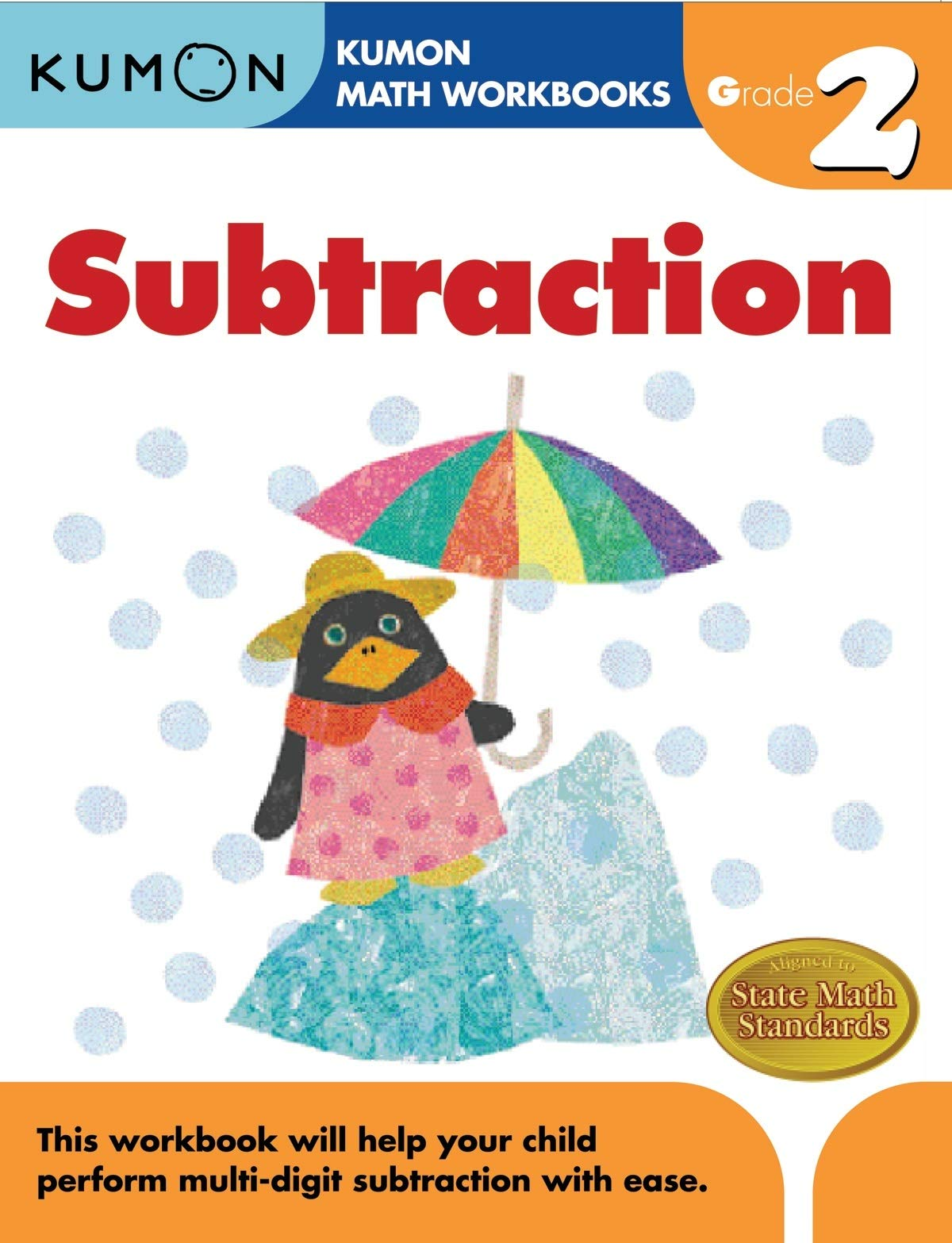Kumon